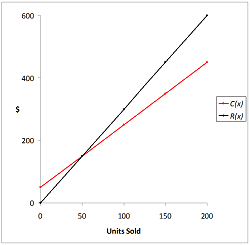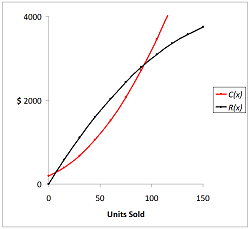# Profit Function: Equation & Formula

Lesson Transcript
Instructor: David Liano

David has a Master of Business Administration, a BS in Marketing, and a BA in History.

This lesson will tell you how to write and use a special type of function called a profit function. After completing this lesson, you will also be able to evaluate profit functions and use them in real life scenarios.

## Definition of a Function

Before we show the profit function equation, we should first make sure that we understand what a general function is. It might be best to think of a function as some type of machine on a factory floor. We enter raw material (input) into the machine, then the machine processes the material, and finally, it creates a product (output).

The input is the domain of the function and the output is the range of the function. The domain is usually represented by the variable x and it is called the independent variable. Each value used for the independent variable produces an output value that is unique to the independent variable. In other words, each input has only one output. The output, or range, of a function is often represented by the variable y.

An error occurred trying to load this video.

Try refreshing the page, or contact customer support.

Coming up next: Abstract Equation: Definition & Concept

### You're on a roll. Keep up the good work!

Replay
Your next lesson will play in 10 seconds
• 0:01 Definition of a Function
• 0:54 Function Notation
• 2:15 Profit Function Equation
• 7:30 Marginal Revenue and…
• 9:55 Lesson Summary
Save Save

Want to watch this again later?

Timeline
Autoplay
Autoplay
Speed Speed

## Function Notation

An example of a function could be y = 3x + 7. We can replace y with the symbol f(x) - f(x) = 3x + 7. The symbol f(x) can be read as 'f of x' or as 'the value of f at x'. In this example, the name of the function is f. If we want to evaluate this function when x = 8, we would complete the following calculation:

f(8) = 3(8) + 7
f(8) = 24 + 7
f(8) = 31

We can use other letters to name functions and other letters to represent the input variable. Let's say that Tom created a function that determines his salary for each week. Therefore, he named this function s. His weekly salary is based on the number of hours he works and he makes $15.00 per hour. Therefore, he used the letter h for his input or independent variable. His function is as follows: s(h) = 15h This is a very simple function, but the main point here is that we can use alternate variables that make sense relevant to the purpose of a function. ## Profit Function Equation A profit function is a function that focuses on business applications. The primary purpose for a business is to sell a product or service in order to make a profit, which is the revenue a company receives for selling a product or service less the cost for creating a product or service. The profit function equation is made up of two primary functions: the revenue function and the cost function. If x represents the number of units sold, we will name these two functions as follows: R(x) = the revenue function; C(x) = the cost function. Therefore, our profit function equation will be as follows: P(x) = R(x) - C(x). ## Examples Let's show a simple example. A street vendor in New York City sells hot dogs for$3.00 each. Therefore, his revenue function is R(x) = 3x. His fixed cost for maintaining his stand each day is $50.00 and his variable cost, or the materials needed to make the hot dogs, is$2.00 per hot dog sold. Therefore, his daily cost function is C(x) = 50 + 2x.

We first would like to find how many hot dogs the vendor needs to sell in order to break even. The breakeven point is when revenue equals cost or when the profit is zero. P(x) = R(x) - C(x) = 0. If the profit is zero, then R(x) = C(x). We will use this equation to find the breakeven point - R(x) = C(x). 3x = 50 + 2x. x = 50.

So, the hot dog vendor needs to sell at least 50 hot dogs on a given day to break even. This is also reflected in the graph shown in Figure 1. We will graph the revenue and cost functions instead of the profit function because this strategy will better explain the dynamics of the profit function.The vendor has a positive profit once he sells more than 50 hot dogs in a given day, and he adds \$1.00 to this profit with each hot dog sold over 50. Our profit equation and the graph show that the vendor's profit potential is endless. However, that is not realistic. There are only so many hours in a day and other factors can come into play, such as price discounts when street traffic is low or the spoiling of materials that are not used. Revenue and cost functions are rarely simple linear functions.

Now let's look at a more complex example.

A small business has the following weekly cost and revenue functions for a new product:

C(x) = 200 + 10x + 0.2x^2
R(x) = 40x - 0.1x^2These functions are reflected in the graph shown in Figure 2. You might notice that the slope of the cost function increases as units sold increases. This could be caused by some factor such as the need for overtime to make the higher number of units. You might also notice that the slope of the revenue function decreases as units sold increases. This could be caused by some factor, such as the giving of price discounts for higher sales volumes.

Let's find the company's profit when it sells 75 units in a week. P(x) = R(x) - C(x). P(x) = 40x - 0.1x^2 - (200 + 10x + 0.2x^2). It might be easier to evaluate the revenue and cost functions separately:

R(75) = 40(75) - 0.1(75)^2 = 3000 - 562.5 = 2437.5
C(75) = 200 + 10(75) + 0.2(75)^2 = 200 + 750 + 1125 = 2075
P(75) = 2437.5 - 2075 = 362.50

To unlock this lesson you must be a Study.com Member.

### Register to view this lesson

Are you a student or a teacher?